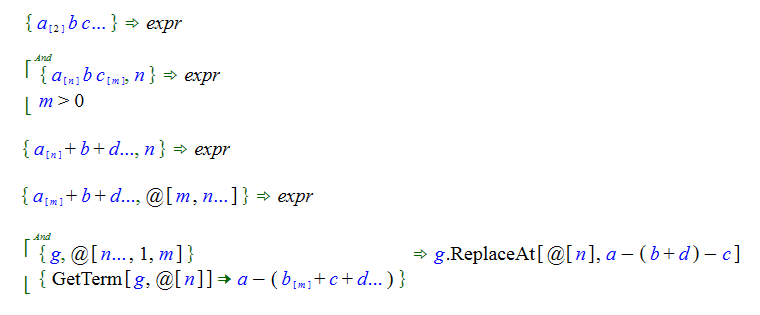# The ListVariableIndexed Object

The ListVariable object is used to bind to an arbitrary number of terms from a list object. The ListVariableIndexed object is similar, but is designed to do one of two things. It can be used to bind to a specified number of parameters of an object or it can be used to return the number of parameters that were bound

In the first case, the index parameter of the ListVariableIndexed object is a specific number. For example a_. Any expression that evaluates to an numeric integer value can also also be used

In the second case, the index parameter of the ListVariableIndexed object is a variable. In this case, the count of the parameters bound to the main variable is included as an additional binding as the value of the variable in the second parameter.

The ListVariableIndexed object has a Standard rendering with the index as a subscript inside square brackets.In each of the examples that follow, the ListVariableIndexed object is included in the pattern and used in a different way:In the first example, "a", "b" and "c" are multiplied together. The first term, containing "a", is a ListVariableIndexed object, the third term, containing "c" is a ListVariable object. This pattern will bind against any Mult object with at least three parameters. The first two parameters will bind to "a", the third parameter will bind to "b", and any additional parameters will be bound to "c". In the case of exactly three parameters, "c" will be bound to the Mult object with no parameters.

In the second example there are two parameters passed into the function. The "a" variable will be bound to a Mult object containing the number of terms specified in the second parameter. The "c" variable will be bound to the remaining terms, as in the previous example, but the "m" variable will also be bound to the number of terms in "c".

In the third example, there are again two parameters. In this case the pattern is an Add object rather than multiplication. The "a" variable will be bound to the number of terms specified in the second parameter. If the second parameter isn't an integer or if it's not consistent with the number of parameters of the first term, then the match will fail.

In the fourth example, we have an expression and a location. Because we are binding "m" terms to the "a" variable, the "b" variable contains the term identified by the location provided in the second parameter.

The last example is a clause from the SignExpression function. The two parameters passed into the function are an expression and the location of the highlight in the expression.

Note that you can use expressions in the index term of a ListVariableIndexed object. For example, you can use something like "n + 1" in the second parameter of a ListVariableIndexed object provided that the variable "n" is already bound.